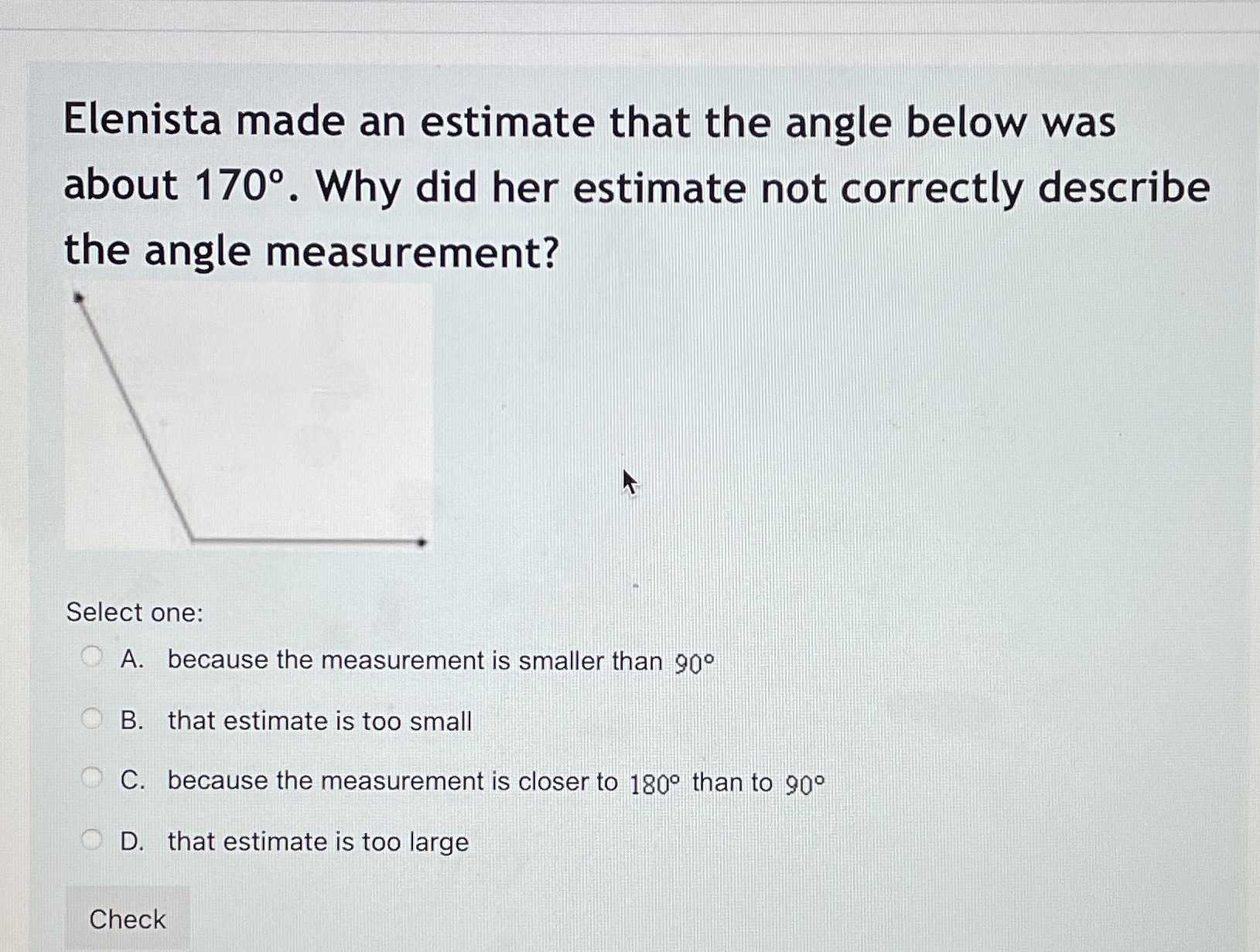### ¿Todavía tienes preguntas de matemáticas?

Pregunte a nuestros tutores expertos
Trigonometry
PreguntaElenista made an estimate that the angle below was about $$170 ^ { \circ }$$ . Why did her estimate not correctly describe the angle measurement? Select one: A. because the measurement is smaller than $$90 ^ { \circ }$$

B. that estimate is too small C. because the measurement is closer to $$180 ^ { \circ }$$ than to $$90 ^ { \circ }$$

D. that estimate is too large